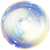# Asynchronous JavaScript Tipsender minyard
💻

To define a synchronous function, you have a few options:

``````let myFunc = () => {...}

function myFunction(){
...
}
``````

Defining function expressions and arrow functions asynchronously looks somewhat different:

``````
let myFunc = async () => {...}

async function myFunction {
...
}
``````

Understanding `await` has helped me a lot.

``````[rv] = await expression;
``````

expression: The promise you're waiting for.

rv: The fulfilled value of the promise.

(You can only use await inside asynchronous functions.)

If you have a promise, this code will fail:

``````let p1 = Promise.resolve(3);
console.log(p1);
``````

But this code will not.

``````
let p1 = Promise.resolve(3);

let myFunc = async () => {
let resolved = await p1;
console.log(resolved); //3
}

myFunc(); // You also could have used an IIFE
``````

It's also useful (and fun!) to understand promises.